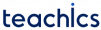# Requirements of a Sensor

The basic requirements of a sensor are

1. Accuracy: It is the level of exactness between the measured value and the true value.
2. Hysteresis: It is the difference in the output of the sensor for a given input x when x reaches this value in upscale direction and downscale direction.
3. Linearity: It is specified in terms of percentage of non-linearity. Non-linearity is the deviation of a sensor output value (real value) from its ideal output value.
4. Range: It shows the minimum and maximum limits in which the input can vary.
5. Repeatability: Repeatability is the capacity of a sensor to deliver the same output for various applications without changing the input value.
6. Response Time: It shows how fast the output changes on a stepwise change in input.
7. Ruggedness: It shows how durable a sensor is when operated under extreme conditions.
8. Sensitivity: It is the ratio of incremental output to incremental input
Subscribe
Notify of